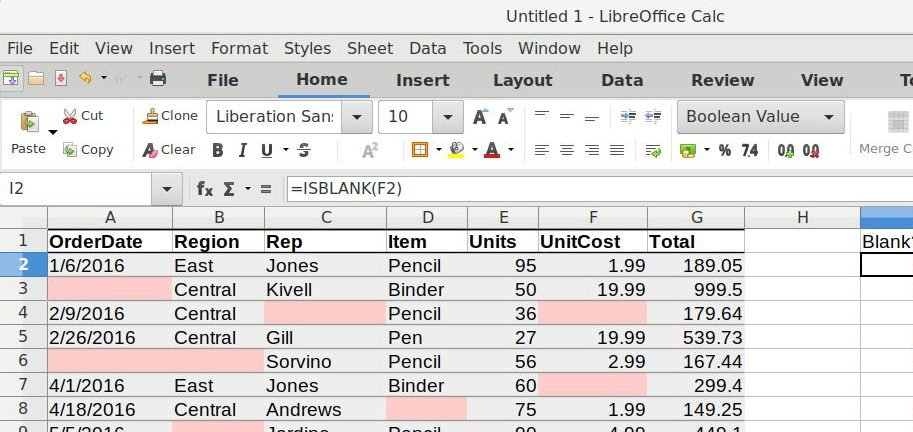## Check Multiple Conditions Using IFS Function in LibreOffice Calc

This guide explains how you can check for multiple conditions in Calc using IFS function.

## Generic Formula

`=IFS(test1, result1, [test2, result2],...)`

test1: First logical test

result1: result when test1 is true.

test2, result2 [optional] – second test value pair.

## Explanation of IFS Function

When you need to check for multiple conditions in a single Calc cell, you should use the IFS function. For example, we have a cell having marks and we have to determine the grade for it based on a certain range of marks as below.

You can find out the grade of any marks based on a series of conditions. For example, if your mark is 55, you can try out the below formula to find out the grade.

`=IFS(E5<40,"D",E5<60,"C",E5<80,"B",E5>=80,"A")`

Similarly, you can change the above formula to find out marks by changing guideline grades.

## Notes on IFS Function

• There is no way to set a default value in IFS if all the conditions are either TRUE or FALSE. In those cases, keep the last condition test as TRUE or FALSE hard-coded and return a value.
• If all the conditions are FALSE, then IFS returns #N/A error.